GeeksforGeeks App
Open AppBrowser
Continue

## Related Articles

• RD Sharma Class 10 Solutions

# Class 10 RD Sharma Solutions – Chapter 4 Triangles – Exercise 4.7 | Set 2

### Question 15. Each side of a rhombus is 10 cm. If one of its diagonals is 16 cm, find the length of the other diagonal.

Solution: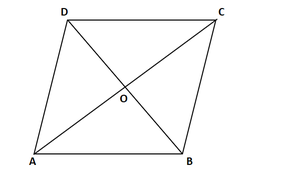Given,

In rhombus ABCD, diagonals AC and BD bisect each other at O at right angles

Each side = 10 cm and one diagonal AC = 16 cm

∴ AO = OC = 16/2 = 8 cm

Now in ∆AOB,

By using Pythagoras theorem

AB2 = AO2 + OB2

(10)2 = (8)2 + (BO)2

100 = 64 + BO2

BO2 = 100 – 64 = 36

BO = 6

So, BD = 2BO = 2 x 6 = 12 cm

Hence, the length of the other diagonal is 12 cm

### Question 16. Calculate the height of an equilateral triangle each of whose sides measures 12 cm.

Solution: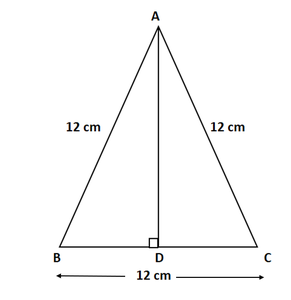Given,

Side of equilateral triangle=12 cm

To find: Calculate the height of an equilateral triangle

Let us draw the figure. Let us draw the altitude AD.

BD = DC = 6cm               [ Altitude is also median of the equilateral triangle]

By using Pythagoras theorem

AD2 = 144 − 36 = 108

Hence, the height of the equilateral triangle is 10.39 cm

### (ii) b2 = a2 + c2 – 2ax

Solution:Given : In ∆ABC, ∠B < 90°

AD = c, BC = a, CA = b AD = h, BD = x, DC = a – x

By using Pythagoras theorem

b2 = h2 + (a – x)2

So, b2 = h2 + a2 + x2 – 2ax

By using Pythagoras theorem

c2 = h2 + x2       …..….(i)

b2 = h2 + a2 + x2 – 2ax

= h2 + x2 + a2 – 2ax

= c2 + a2 – 2ax         [From eq(i)]

So, b2 = a2 + c2 – 2ax

Hence proved.

### Question 18. In an equilateral ∆ABC, AD ⊥ BC, prove that AD2 = 3 BD2.

Solution: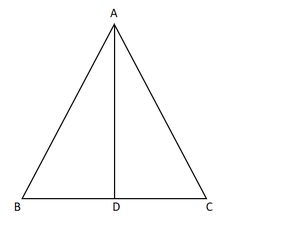In right-angled ∆ABD,

By using Pythagoras theorem

AB2 = AD2 + BD2     ….(1)

We know that in an equilateral triangle every altitude is also median.

We have BD = DC

Since ∆ABC is an equilateral triangle, AB = BC = AC

So, we can write equation (1) as

BC2 = AD2 + BD2       ….(2)

But BC = 2BD

Therefore, equation (2) becomes,

On simplifying the equation we get,

Hence proved

### Question 19. ∆ABD is a right triangle right-angled at A and AC ⊥ BD. Show that

(i) AB2 = BC.BD

(ii) AC2 = BC.DC

(iv) AB2/AC2 = BD/DC

Solution: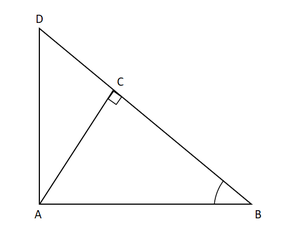∠DAB = ∠ACB = 90°

∠ABD = ∠CBA                       (common angle)

So, by AAA

Hence,

AB/CB = BD/AB

⇒ AB2 = CB × BD

(ii) Let ∠CAB = y

In ΔCBA

∠CBA = 180° − 90° − y

∠CBA = 90° − y

∠CDA = 90° − ∠CAB

= 90° − y

∠CDA = 180° −90° − (90° − y)

∠CDA = y

∠CAB = ∠CDA

∠ACB = ∠DCA = 90°

So, by AAA rule

So, AC /DC = BC/AC

⇒ AC2 = DC × BC

(iii) In ΔDCA & ΔDAB

∠DCA = ∠DAB     (both are equal to 90°)

∠DAC = ∠DBA     (remaining angle)

So, by AAA rule

ΔDCA ~ ΔDAB

so, DC/DA = DA/DB

⇒ AD2 = BD × CD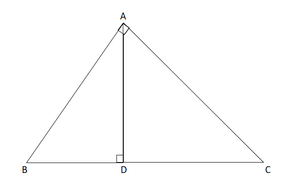(iv) From part (i) AB2 = CB x BD

From part (ii) AC2 = DC × BC

So, AB2/AC2= CB x BD/DC x BC

AB2/AC2 = BD/DC

Hence proved

### Question 20. A guy wire attached to a vertical pole of height 18 m is 24 m long and has a stake attached to the other end. How far from the base of the pole should the stake be driven so that the wire will be taut?

Solution: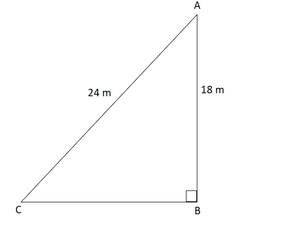Given,

AC = 18m be the height of pole.

BC = 24m is the length of a guy wire and is attached to stake B.

Now,

In △ABC

By using Pythagoras theorem

BC2 = AB2 + AC2

242 = AB2 + 182

AB2 = 576 − 324

= 252

So, AB = 6√7 m

Hence, the stake has to be 6√7 m from base A.

### Question 21. Determine whether the triangle having sides (a – 1) cm, 2 √a cm and (a + 1) cm is a right-angled triangle.

Solution: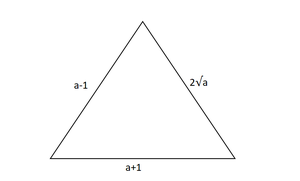Given,

The sides of triangle are (a – 1) cm, 2√a and (a + 1) cm.

Let us considered ABC be the triangle in which with the sides are

AB = (a – 1)cm, BC = (2√ a) cm, CA = (a + 1) cm

AB² = (a – 1)

By using (a – b)2 = a2 + b2 – 2ab

= a2 + 12 – 2 × a × 1

AB2 = a2 + 1 -2a

BC2 = (2√a)2

∴ BC = 4a

CA2 = (a + 1)2

By using (a + b)2 = a2 + b2 + 2ab

= a2 + 12 + 2 × a × 1

CA2 = a2+ 1 + 2a

By using Pythagoras Theorem

AC2 = AB2 + BC2

On putting value of AC2, AB2 and BC2 in the above equation,

a2 + 1 + 2a = a2 + 1 – 2a + 4a

a2 + 1 + 2a = a2 + 1 + 2a

AC2 = AB2 + BC2

∆ABC is right-angled ∆ at B.

Hence proved

### Question 22. In an acute-angled triangle, express a median in terms of its sides.

Solution: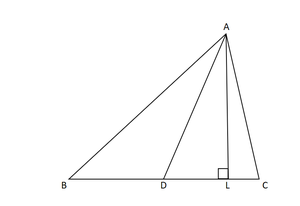Given,

In Δ ABC AD is median.

Construction: AE ⊥ BC

Now,

∴ BD = CD = 1/2 BC ….(1)         [AD is the median]

In Δ AED,

By using Pythagoras Theorem

⇒ AE2 = AD2 – DE2 …..(2)

In Δ AEB,

AB2 = AE2 + BE2

⇒ AD2 – DE2 + BE2                       [From eq(2)]

= (BD + DE)2 + AD2 – DE2                [∴ BE = BD + DE]

BD2 + DE2 + 2BD x DE + AD2 – DE2

= BD2 + AD2 + 2BD x DE

= (1/2BC)2 + AD2 + (2 × 1/2BC × DE)   [From eq(1)]

= (1/4BC)2 + AD2 + BC x DE ….(3)

In Δ AED,

By using Pythagoras Theorem

AC2 = AE2 + EC2

= AD2 – DE2 + EC2

= AD2 – DE2 + (DC – DE)2

= AD2 – DE2 + DC2 + DE2 – 2DC x DE

AD2 + DC2 – 2DC x DE

= AD2 + (1/2BC)2 – (2 × 1/2BC x DE)

= AD2 + (1/4BC)2 – BC x DE ….(4)

On adding eq(3) and (4), we get

AB2 + AC2 = 1/4BC2 + AD2 + BC x DE + AD2 + 1/4BC2 – BC x DE

2(AB2 + AC2) = BC2 + 4AD2

2AB2 + 2AC2 = BC2 + 4AD2

Hence Proved

### Question 23. In right-angled triangle ABC in which ∠C = 90°, if D is the mid point of BC, prove that AB2 = 4AD2 – 3AC2.

Solution: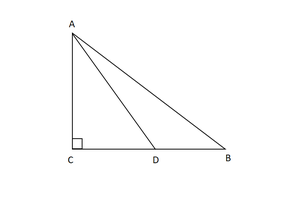Given :

∠C = 90° and D is the mid-point of BC.

To prove : AB2 = 4AD2 – 3AC2

In ∆ ACD,

By using Pythagoras Theorem

CD2 = AD2 – AC2  ……….(1)

In ∆ACB,

By using Pythagoras Theorem

AB2 = AC2 + BC2

AB2 = AC2 + (2CD)2   [D is the mid-point of BC]

AB2 = AC2 + 4CD2

∴ AB2 = AC2 + 4(AD2 – AC2)    [From eq(1)]

AB2 = AC2 + 4AD2 – 4AC2

AB2 = 4AD2 – 4AC2 + AC2

∴ AB2 = 4AD2 – 3AC2

Hence Proved

### Question 24. In the figure, D is the mid-point of side BC and AE ⊥ BC. If BC = a, AC = b, AB = c, ED = x, AD = p and AE = h, prove that

(i) b2 = p2 + a2/4 + ax

(ii) c2 = p2– ax + a2/4

(iii) b2 + c2 = 2p2 + a2/2

Solution: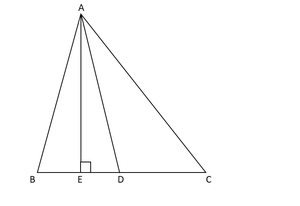Given,

D is the midpoint of BC

(i) In ∆ AEC

AC2 = AE2 + EC2

b2 = AE2 + (ED + DC)2

b2 = AD2 + DC2 + 2 x ED x DC            [Given BC = 2CD]

b2 = p2 + (a/2)2 + 2(a/2)x

b2 = p2 + a2/4 + ax      ………..(i)

(ii) In ∆ AEB

AB2 = AE2 + BE2

c2 = AD2 – ED2 + (BD – ED)2

c2 = p2 – ED2 + BD2 + ED2 – 2BD x ED

c2 = P2 + (a/2)2 – 2(a/2)2x

c2 = p2 – ax + a2/4 ……………….(ii)

(iii) Adding eqn (i) and (ii) we get,

b2 + c2 = 2p2 + a2/2

Hence Proved

### Question 25. In ∆ABC, ∠A is obtuse, PB x AC and QC x AB. Prove that:

(i) AB x AQ = AC x AP

(ii) BC2 = (AC x CP + AB x BQ)

Solution: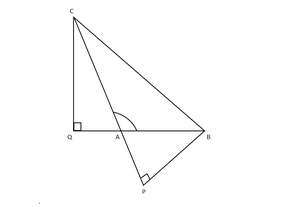(i) Given :

∠A is obtuse.

PB is perpendicular to AC.

QC is perpendicular to AB.

To Prove :

AB × AQ = AC × AP.

Proof:

In ΔACQ and ΔABP,

⇒ ∠CAQ = ∠BAP          [Vertically opposite ∠]

⇒ ∠Q = ∠P                    [∠Q = ∠P = 90 º]

So, by AA rule

ΔACQ ~ ΔABP

By Property of Similar Triangles,

⇒ CQ/BP = AC/AB = AQ/AP

⇒ AC/AB = AQ/AP

⇒ AB × AQ = AC × AP  ……..(i)

Hence Proved.

(ii) To Prove :

BC² = AB × BQ + AC × CP

Proof:

By using Pythagoras Theorem

⇒ BC2 = CQ2 + QB2

⇒ BC2 = CQ2 + (QA + AB)2

⇒ BC2 = CQ2 + QA2 + AB2 + 2 QA × AB

⇒ BC2 = CQ2 + QA2 + AB2 + QA × AB + QA × AB

⇒ BC2 = AC2 + AB2 + QA × AB + QA × AB           [In ΔACQ, CQ2 + QA2 = AC2]

⇒ BC2 = AC2 + AB2 + QA × AB + AC × CP            [By Eq (i)]

⇒ BC2 = AC2 + AC × CP + AB² + QA × AB

⇒ BC2 = AC × (AC + CP) + AB × (QA + AB)

⇒ BC2 = AC × CP + AB × BQ                                  [CP = AC + CP, BQ = AQ + AB]

⇒ BC2 = AB × BQ + AC × CP.

Hence, Proved.

### Question 26. In a right ∆ABC right-angled at C, if D is the mid-point of BC, prove that BC2 = 4 (AD2 – AC2).

Solution: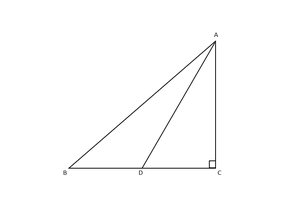Given:

∠C = 90°

By using Pythagoras Theorem

AD2 = AC2 + (1/2BC)2    [DC = 1/2BC]

Taking minus common

Hence, proved

### Question 27. In a quadrilateral ABCD, ∠B = 90°, AD2 = AB2 + BC2 + CD2, prove that ∠ACD = 90°.

Solution: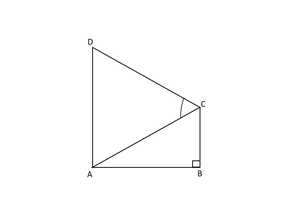Given: ABCD is a quadrilateral, ∠B = 90° and AD2 = AB2 + BC2 + CD2

To prove: ∠ACD = 90°

Proof: In right ∆ABC,

By using Pythagoras Theorem

AC2 = AB2 + BC2 … (1)

Given, AD2 = AB2 + BC2 + CD2

⇒ AD2 = AC2 + CD2  [Using eq(1)]

In ∆ACD,

So, ∠ACD = 90° [By converse of Pythagoras theorem]

### Question 28. An aeroplane leaves an airport and flies due north at a speed of 1000 km/hr. At the same time, another aeroplane leaves the same airport and flies due west at a speed of 1200 km/ hr. How far apart will be the two planes after 1and1/2 hours?

Solution: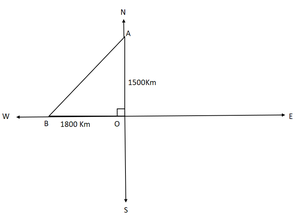Given that speed of first aeroplane = 1000 km/hr

Distance travelled by first aeroplane (due north) inhours = 1000 x 3/2 km = 1500 km

Speed of second aeroplane = 1200 km/hr

Distance travelled by first aeroplane (due west) inhours = 1200 × 3/2 km = 1800 km

Now in ΔAOB,

Using Pythagoras Theorem,

AB2 = AO2 + OB2

⇒ AB2 = (1500)2 + (1800)2

⇒ AB = √(2250000 + 3240000)

= √5490000

⇒ AB = 300√61 km

Hence, inhours the distance between two plane = 300√61 km.

My Personal Notes arrow_drop_up
Related Tutorials